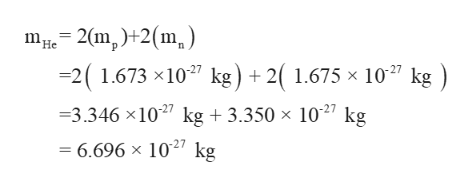# How many electrons would it take to equal the mass of a helium nucleus?

Question
301 views

How many electrons would it take to equal the mass of a helium nucleus?

check_circle

Step 1

The helium atom has 2 protons, 2 neutrons and 2 electrons. The mass of helium nucleus depends only on 2 protons and 2 neutrons. The mass of proton(mp) is 1.673×10-27 kg and the mass of neutron (me)is 1.675 ×10-27 kg. The total mass of nucleus  (mHe) is calculated below.help_outlineImage Transcriptionclose- 2(m,) 2(m) -2( 1.673 x1027 kg) 2(1.675 x 1027 kg ) m He + 1027 kg 3.346 x1027 kg + 3.350 x = 6.696 x 1027 kg fullscreen
Step 2

An electron has a mass (me) of 9.1 ×10-31 kg. The number of electrons (ne)that are equal to the mass of Helium (mHe) is calculated by divi...

### Want to see the full answer?

See Solution

#### Want to see this answer and more?

Solutions are written by subject experts who are available 24/7. Questions are typically answered within 1 hour.*

See Solution
*Response times may vary by subject and question.
Tagged in

### General Chemistry